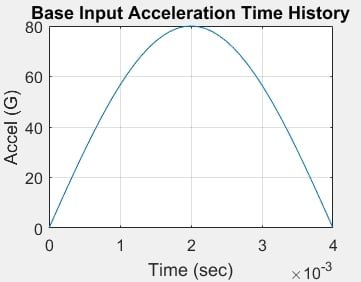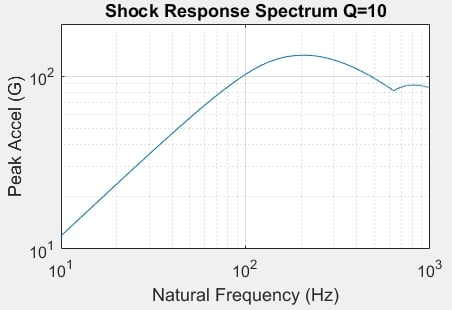## General Mechanical

•Ofekv
Subscriber

Hi everyone

I am trying to simulate a hammer impact test on several bodies.

I have done the modal analysis and have good results, now i want to try adding an impulse to get the response and deformations as well.

Selecting "transient structural" gives an option to add a load,  but it's either constant, or i can limit it to a certain time, but the timesteps are way too big.

Can anyone help and share how to correctly add an impact at a specific point to get the maximum deformations?

Any help would be much appreciated. Thank you.

•peteroznewman
Subscriber

A common way to apply a shock to a structure is through a base input. That means that the Fixed Support you used for the Modal analysis becomes a Base Input to a Modal Superposition (MSUP) Transient analysis. When you add the Transient Structural, drop it on the Solution cell of the Modal analysis and you will be able to define a Base Input.  Note that this is a linear analysis, so you won't be permitted to do this if you have nonlinear materials or nonlinear contacts in the model.

What is the lowest frequency from the modal analysis?  Let's say it was 20 Hz.  If you want to see 10 cycles of that lowest frequency to watch the decay due to damping, then your End Time would have to be 10/20 = 0.5 seconds.

What is the highest frequency you want to resolve in your simulation?  Let's say it is 250 Hz.  You need at least 20 time steps to resolve a 250 Hz frequency. That means a time step not larger than 1/(20*250) = 0.0002 s time steps.  Your simulation will need 2,500 time steps to get to 0.5 seconds of simulated time. That is a lot of data.  Make sure you have a large local HDD (or SSD) to store all that data.

It's important to create a base input acceleration that has the frequency content that can excite the highest frequency of interest.  For example, use a half sine waveform and reach a peak of 80 G with a duration of 4 ms as shown below. If 80 G seems too high, just reduce it to a lower level like 10 G. Note that you can't input data in units of G to ANSYS. You have to convert that to m/s^2.The Shock Response Spectrum for the above half sine is shown below. Note that the peak response is around 200 Hz.Create a spreadsheet with two columns, time and acceleration magnitude in m/s^2.  You can make the acceleration profile what you want.  A good choice would be a half sine that starts at 0.0, 0.0 rises to a maximum, then returns to zero at the duration you want for the impulse, for example, 0.004 s. Make the delta T so that there are at least 10 increments to the duration you want. That would be 0.0004 s time steps in this example. Since this value is more than the 0.0002 s calculated above, use 0.0002 s for all the time steps.

Add an Acceleration load and set Base Excitation to Yes, Define by Vector (assuming you have a face or edge to set the direction of the shock) the under Magnitude, select Tabular data, finally, copy the two columns from Excel and paste them into the Table. Make sure the units in ANSYS are set to SI so that the values you paste in are in m/s^2 and match the units in ANSYS. After the row that has 0.004, 0.0 which is the last row of the half sine, the next row in the table can be the end time of 0.5, 0.0.  You don't need a row for each time step.

If you really want a hammer strike on a particular body, and not a Base Acceleration, you can apply a Force load with a profile the same as above, but in the units of Newton, using the same method described above, but select a face to scope the force load.  If the face available is too large, go into the Geometry editor and divide out a small square or circle to apply the load to that small face. Don't apply the Force to a vertex on a solid model, that will cause a high stress on that element.